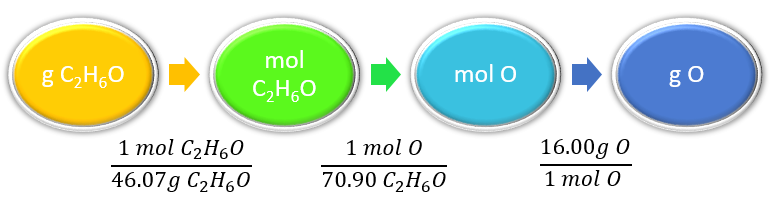# 6.5: Chemical Formulas as Conversion Factors

Learning Objectives

• Use chemical formulas as conversion factors.

Figure $$\PageIndex{1}$$ shows that we need 2 hydrogen atoms and 1 oxygen atom to make one water molecule. If we want to make two water molecules, we will need 4 hydrogen atoms and 2 oxygen atoms. If we want to make five molecules of water, we need 10 hydrogen atoms and 5 oxygen atoms. The ratio of atoms we will need to make any number of water molecules is the same: 2 hydrogen atoms to 1 oxygen atom.Figure $$\PageIndex{1}$$ Water Molecules. The ratio of hydrogen atoms to oxygen atoms used to make water molecules is always 2:1, no matter how many water molecules are being made.

Using formulas to indicate how many atoms of each element we have in a substance, we can relate the number of moles of molecules to the number of moles of atoms. For example, in 1 mol of water (H2O) we can construct the relationships given in (Table $$\PageIndex{1}$$).

Table $$\PageIndex{1}$$: Molecular Relationships for Water
1 Molecule of $$H_2O$$ Has 1 Mol of $$H_2O$$ Has Molecular Relationships
2 H atoms 2 mol of H atoms $$\mathrm{\dfrac{2\: mol\: H\: atoms}{1\: mol\: H_2O\: molecules}}$$ or $$\mathrm{\dfrac{1\: mol\: H_2O\: molecules}{2\: mol\: H\: atoms}}$$
1 O atom 1 mol of O atoms $$\mathrm{\dfrac{1\: mol\: O\: atoms}{1\: mol\: H_2O\: molecules}}$$ or $$\mathrm{\dfrac{1\: mol\: H_2O\: molecules}{1\: mol\: O\: atoms}}$$

The Mole is big

A mole represents a very large number! The number 602,214,129,000,000,000,000,000 looks about twice as long as a trillion, which means it’s about a trillion trillion.(CC BY-SA NC; https://what-if.xkcd.com/4/).

A trillion trillion kilograms is how much a planet weighs. If 1 mol of quarters were stacked in a column, it could stretch back and forth between Earth and the sun 6.8 billion times.

Table $$\PageIndex{2}$$: Molecular and Mass Relationships for Ethanol
1 Molecule of $$C_2H_6O$$ Has 1 Mol of $$C_2H_6O$$ Has Molecular and Mass Relationships
2 C atoms 2 mol of C atoms $$\mathrm{\dfrac{2\: mol\: C\: atoms}{1\: mol\: C_2H_6O\: molecules}}$$ or $$\mathrm{\dfrac{1\: mol\: C_2H_6O\: molecules}{2\: mol\: C\: atoms}}$$
6 H atoms 6 mol of H atoms $$\mathrm{\dfrac{6\: mol\: H\: atoms}{1\: mol\: C_2H_6O\: molecules}}$$ or $$\mathrm{\dfrac{1\: mol\: C_2H_6O\: molecules}{6\: mol\: H\: atoms}}$$
1 O atom 1 mol of O atoms $$\mathrm{\dfrac{1\: mol\: O\: atoms}{1\: mol\: C_2H_6O\: molecules}}$$ or $$\mathrm{\dfrac{1\: mol\: C_2H_6O\: molecules}{1\: mol\: O\: atoms}}$$

2 (12.01 amu) C

24.02 amu C

2 (12.01 g) C

24.02 g C

$$\mathrm{\dfrac{24.02\: g\: C\: }{1\: mol\: C_2H_6O\: molecules}}$$ or $$\mathrm{\dfrac{1\: mol\: C_2H_6O\: molecules}{24.02\: g\: C\: }}$$

6 (1.008 amu) H

6.048 amu H

6 (1.008 g) H

6.048 g H

$$\mathrm{\dfrac{6.048\: g\: H\: }{1\: mol\: C_2H_6O\: molecules}}$$ or $$\mathrm{\dfrac{1\: mol\: C_2H_6O\: molecules}{6.048\: g\: H\: }}$$

1 (16.00 amu) O

16.00 amu O

1 (16.00 g) O

16.00 g O

$$\mathrm{\dfrac{16.00\: g\: O\: }{1\: mol\: C_2H_6O\: molecules}}$$ or $$\mathrm{\dfrac{1\: mol\: C_2H_6O\: molecules}{16.00\: g\: O\: }}$$

The following example illustrates how we can use the relationships in Table $$\PageIndex{2}$$ as conversion factors.

Example $$\PageIndex{1}$$: Ethanol

If a sample consists of 2.5 mol of ethanol (C2H6O), how many moles of carbon atoms does it have?

Solution

Steps for Problem Solving

If a sample consists of 2.5 mol of ethanol (C2H6O), how many moles of carbon atoms does it have?

Identify the "given" information and what the problem is asking you to "find."

Given: 2.5 mol C2H6O
Find: mol C atoms

List other known quantities.

1 mol C2H6O = 2 mol C

Prepare a concept map and use the proper conversion factor.Cancel units and calculate.

Note how the unit mol C2H6O molecules cancels algebraically.

$$\mathrm{2.5\: \cancel{mol\: C_2H_6O\: molecules}\times\dfrac{2\: mol\: C\: atoms}{1\: \cancel{mol\: C_2H_6O\: molecules}}=5.0\: mol\: C\: atoms}$$

Think about your result. There are twice as many C atoms in one C2H6O molecule, so the final amount should be double.

Exercise $$\PageIndex{1}$$

If a sample contains 6.75 mol of Na2SO4, how many moles of sodium atoms, sulfur atoms, and oxygen atoms does it have?

13.5 mol Na atoms, 6.75 mol S atoms, and 27.0 mol O atoms

The fact that 1 mol equals 6.022 × 1023 items can also be used as a conversion factor.

Example $$\PageIndex{2}$$: Oxygen Mass

Determine the mass of Oxygen in 75.0g of C2H6O.

Solution

Steps for Problem Solving

Determine the mass of Oxygen in 75.0g of C2H6O

Identify the "given" information and what the problem is asking you to "find."

Given: 75.0g C2H6O
Find: g O

List other known quantities.

1 mol O = 16.0g O

1 mol C2H6O = 1 mol O

1 mol C2H6O = 46.07g C2H6O

Prepare a concept map and use the proper conversion factor.Cancel units and calculate.

$$\require{cancel}\mathrm{75.0\: \cancel{g\: C_2H_6O}\times\dfrac{1\: \cancel{mol\: C_2H_6O}}{46.07\:\cancel{g\: C_2H_6O}}\times\dfrac{1\: \cancel{mol\:O}}{1\: \cancel{mol\:C_2H_6O}}\times\dfrac{16.00\: g\: O}{1\: \cancel{mol\:O}}=26.0\: g\: O}$$

Exercise $$\PageIndex{2}$$

1. How many molecules are present in 16.02 mol of C4H10? How many C atoms are in 16.02 mol?
2. How many moles of each type of atom are in 2.58 mol of Na2SO4?